# Chemical Engineering Thermodynamics Questions and Answers

## Multiple Choice Questions (MCQ) and Answers on Chemical Engineering Thermodynamics

Question 1 : The co-efficient of performance (COP) of a refrigerating system, which is its index of performance, is defined as the ratio of useful refrigeration to the net work. The units of __________ and COP are the same.

1. kinematic viscosity
2. work
3. temperature
4. none of these

Question 2 : The chemical potential of a component (μi) of a phase is the amount by which its capacity for doing all work, barring work of expansion is increased per unit amount of sustance added for an infinitesimal addition at constant temperature and pressure. It is given by

1.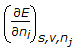2.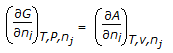3.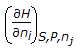4. all (1), (2) and (3)

Question 3 : In jet refrigerators, the refrigerating fluid is practically always

1. water
2. ammonia
3. freon
4. brine

Question 4 : Water on heating from 1 to 4°C

1. contracts
2. expands
3. has same volume
4. may contract or expand

Question 5 : Pick out the correct statement.

1. Compression ratio of an Otto engine is comparatively higher than a diesel engine.
2. Efficiency of an Otto engine is higher than that of a diesel engine for the same compression ratio.
3. Otto engine efficiency decreases with the rise in compression ratio, due to decrease in work produced per quantity of heat.
4. Diesel engine normally operates at lower compression ratio than an Otto engine for an equal output of work.

Question 6 : Equilibrium constant decreases as the temperature

1. increases, for an exothermic reaction.
2. decreases, for an exothermic reaction.
3. increases, for an endothermic reaction.
4. none of these.

Question 7 : The following expression,  , gives the free energy change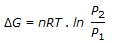1. with pressure changes at constant temperature.
2. under reversible isothermal volume change.
3. during heating of an ideal gas.
4. during cooling of an ideal gas.

Question 8 : All gases during throttling process at atmospheric temperature and pressure show a cooling effect except

1. CO2
2. H2
3. O2
4. N2

Question 9 : Compressibility factor for almost all the gases are approximately same at the same

1. pressure and temperature.
2. reduced pressure and reduced temperature.
3. critical pressure and critical temperature.
4. none of these.

Question 10 : Pick out the wrong statement.

1. Activity co-efficient is dimensionless.
2. In case of an ideal gas, the fagacity is equal to its pressure.
3. In a mixture of ideal gases, the fugacity of a component is equal to the partial pressure of the component.
4. The fugacity co-efficient is zero for an ideal gas.

Question 11 : In a reversible chemical reaction (where, Δx = number of moles of products-number of moles of reactants )

1. addition of inert gas favours the forward reaction, when Δx is positive.
2. pressure has no effect on equilibrium, when Δn = 0.
3. addition of inert gas has no effect on the equilibrium constant at constant volume for any value of Δx (+ ve, – ve) or zero).
4. all ‘1’, ‘2’ & ‘3’.

Question 12: Out of the following refrigeration cycles, which one has maximum COP ?

1. Air cycle
2. Carnot cycle
3. Ordinary vapor compression cycle
4. Vapor compression with a reversible expansion engine

Question 13 : Pick out the correct statement:

1. In an isothermal system, irreversible work is more than reversible work.
2. Under reversible conditions, the adiabatic work is less than isothermal work.
3. Heat, work, enthalpy and entropy are all ‘state functions’.
4. Matter and energy can not be exchanged with the surroundings in a closed system.

Question 14 : In any spontaneous process,

1. only F decreases
2. only A decreases
3. both F and A decreases
4. both F and A increase

Question 15 : Which of the following is a thermodynamic property of a system ?

1. Concentration
2. Mass
3. Temperature
4. Entropy

Question 16 : Linde gas liquefaction process employs cooling

1. by throttling
2. by expansion in an engine
3. at constant pressure
4. none of these

Question 17 : Pick out the wrong statement pertaining to the decomposition of PCl5 represented by, PCl5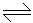PCl3 + Cl2.Degree of dissociation of PCl5 will

1. decrease on addition of Cl2.
2. increase on addition of an inert gas at constant pressure.
3. decrease on increasing the pressure of the system.
4. none of these

Question 18 : Joule-Thomson experiment is

1. isobaric
3. isenthalpic
4. both(2) & (3)

Question 19 : Boyle’s law for gases states that

1. P ∝ 1/V, when temperature is constant.
2. P ∝ 1/V, when temperature & mass of the gas remain constant.
3. P ∝ V, at constant temperature & mass of the gas.
4. P/V =  constant, for any gas

Question 20 : 1st law of thermodynamics is nothing but the law of conservation of

1. momentum
2. mass
3. energy
4. none of these

Question 21 : “At the absolute zero temperature, the entropy of every perfectly crystalline substance becomes zero”. This follows from the

1. third law of thermodynamics
2. second law of thermodynamics
3. Nernst heat theorem
4. Maxwell’s relations

Question 22 : During Joule-Thomson expansion of gases

1. enthalpy remains constant.
2. entropy remains constant.
3. temperature remains constant.
4. none of these.

Question 23 : The accentric factor of a materical, ‘ω’, is defined as ω = -log10(Prsat)Tr-1 = 0.7, where, Prsat = reduced vapor pressure, Tr = reduced temperature. The value of accentric factor is always

1. >2
2. <1
3. >1
4. <3

Question 24 : Degress of freedom at triple point will be

1. 0
2. 1
3. 2
4. 3

Question 25 : “Law of corresponding states” says that

1. two different gases behave similarly, if their reduced properties (i.e. P, V and T) are same.
2. the surface of separation (i. e. the meniscus) between liquid and vapour phase disappears at the critical temperature.
3. no gas can be liquified above the critical temperature, howsoever high the pressure may be.
4. the molar heat of energy of gas at constant volume should be nearly constant (about 3 calories).

Question 26 : Entropy of an ideal gas depends upon its

1. pressure
2. temperature
3. both (1) & (2)
4. neither (1) nor (2)

Question 27 : Isobaric process means a constant process.

1. temperature
2. pressure
3. volume
4. entropy

Question 28: No work is done by the system, when a reaction occurs at constant

1. volume
2. temperature
3. pressure
4. none of these

Question 29 : If the vapour pressure at two temperatures of a solid phase in equilibrium with its liquid phase are known, then the latent heat of fusion can be calculated by the

1. Maxwell’s equation
2. Clayperon-Claussius equation
3. Van Laar equation
4. Nernst Heat Theorem

Question 30 : The freezing point of a liquid decreases when the pressure is increased, if the liquid __________ while freezing.

1. contracts
2. expands
3. does not change in volume
4. either (1), (2) or (3)

Question 31 : Which of the following is not an intensive property ?

1. Chemical potential
2. Surface tension
3. Heat capacity
4. None of these

Question 32 : Out of the following refrigration cycles, which one has the minimum COP (Co-efficient of performance)?

1. Air cycle
2. Carnot cycle
3. Ordinary vapour compression cycle
4. Vapour compression with a reversible expansion engine

Question 33 : Measurement of thermodynamic property of temperature is facilitated by __________ law of thermodynamics.

1. 1st
2. zeroth
3. 3rd
4. none of these

Question 34 : The theoretical minimum work required to separate one mole of a liquid mixture at 1 atm, containing 50 mole % each of n- heptane and n- octane into pure compounds each at 1 atm is

1. -2 RT ln 0.5
2. RT ln 0.5
3. 0.5 RT
4. RT

Question 35 : Keeping the pressure constant, to double the volume of a given mass of an ideal gas at 27°C, the temperature should be raised to __________ °C.

1. 270
2. 327
3. 300
4. 540

Question 36 : A refrigeration cycle is a reversed heat engine. Which of the following has the maximum value of the co-efficient of performance (COP) for a given refrigeration effect ?

1. Vapor compression cycle using expansion valve.
2. Air refrigeration cycle.
3. Vapor compression cycle using expansion engine.
4. Carnot refrigeration cycle.

Question 37 : In a working refrigerator, the value of COP is always

1. 0
2.  <0
3.  <1
4.  >1

Question 38 : _________ increases with increase in pressure.

1. The melting point of wax
2. The boiling point of a liquid
3. both (1) and (2)
4. neither (1) nor (2)

Question 39 : A refrigerator may be termed as a

1. heat pump
2. heat engine
3. Carnot engine
4. none of these

Question 40 : For a stable phase at constant pressure and temperature, the fugacity of each component in a binary system __________ as its mole fraction increases.

1. decreases
2. increases
3. remains same
4. decreases linearly

Question 41 : Solubility of a substance which dissolves with an increase in volume and liberation of heat will be favoured by the

1. low pressure and high temperature.
2. low pressure and low temperature.
3. high pressure and low temperature.
4. high pressure and high temperature.

Question 42 : Entropy is a measure of the __________ of a system.

1. disorder
2. orderly behaviour
3. temperature changes only
4. none of these

Question 43 : A chemical reaction will occur spontaneously at constant pressure and temperature, if the free energy is

1. zero
2. positive
3. negative
4. none of these

Question 44 : Fundamental principle of refrigeration is based on the __________ law of thermodynamics.

1. zeroth
2. first
3. second
4. third

Question 45 : For a spontaneous process, free energy

1. is zero
2. increases
3. decreases whereas the entropy increases
4. and entropy both decrease

Question 46 : Maximum work that could be secured by expanding the gas over a given pressure range is the __________ work.

1. isothermal
3. isentropic
4. none of these

Question 47 : Internal energy change of a system over one complete cycle in a cyclic process is

1. zero
2. +ve
3. -ve
4. dependent on the path

Question 48 : Heating of water under atmospheric pressure is an __________ process.

1. isochoric
2. isobaric
4. isothermal

Question 49 : Van Laar equation deals with the activity co-efficients in

1. binary solutions
2. ternary solutions
3. azeotropic mixture only
4. none of these